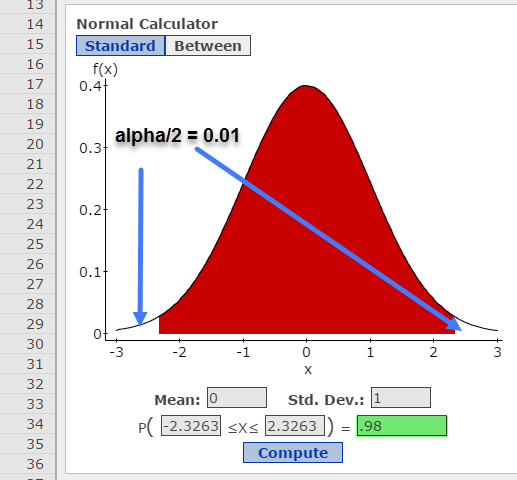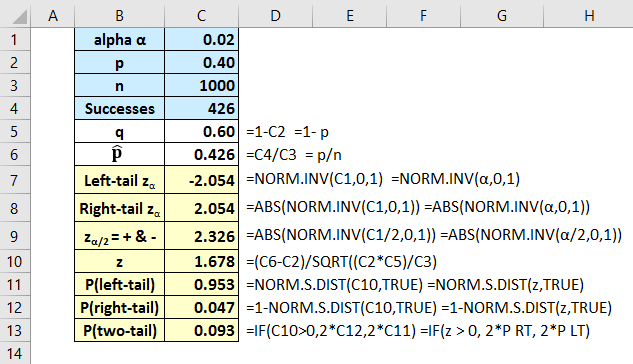# Single-sample Hypothesis Test for a Proportion

Consider the following problem statement:

An article in an online magazine states that 40% of home buyers found their real estate agent through referrals by a friend. However, a professor in a local college sampled 1000 home buyers and found that 426 chose an agent recommended by a friend.

Does the data refute the claim made by the magazine? Use a significance level of 0.02.

Solution:

• First, you should recognize that this is a test about a single proportion, not a mean or other statistic.
• The claim is that the proportion of home buyers who select their real estate agent based on the recommendation of a friend is 0.40. Therefore, the claim is p = 0.40.
• Since the claim contains an equality, =, it must be the null. Ho: p = 0.40.
• The alternative must be the complement, Ha: p ≠ 40.
• Remember the rule of thumb is that all hypothesis tests for proportions are z-tests. But you should confirm that you can use the normal distribution by checking that both n*p and n*q are greater than 5. Here n*p = 2000*0.40 = 800 and n*q = 2000*(1-0.40) = 1200. Both are > 5, there we can use the normal distribution.
• I recommend always sketching the situation described in the problem. Here we see that the sample count of 426 falls on the right side of the hypothesized mean of 400 for the population. Recall, the mean for a proportion is just the n*p or 0.4 * 1000. The standard deviation for a proportion is• Recall, the math operator in the alternative always indicates the tail of the test. In this case, the ≠ math operator indicates this is a two-tailed test.
• With a two-tailed test, you must put half of alpha, α, in each tail. Thus, α/2 = 0.02/2 = 0.010.
• For StatCrunch, we use the Stat > Calculators > Normal command sequence. I like to use the “Between” tool. Here, I entered 1- α = 0.98 in the box (highlighted in green in my image though not in actuality). This is a two-tail test, therefore put α/2 in each tail.Read critical value of z, zα/2  (sometimes shown as z0 ), = -2.33 and +2.33. The rejection regions are in the white areas to the left (less than) -2.33 and to the right (greater than) +2.33.

• We can find the z test statistic using the formula

Because the null is our claim, we conclude: There is insufficient evidence to reject the claim that the proportion of home buyers who find their agents from referrals from friends is 40%.

• I like to use Excel to set up the problem so that I can save and reuse the worksheet on similar problems.• We get the same values for the critical value of z, +/- 2.326, and the test statistic, z = 1.678.
• Remember that the p-value for a two-tail test is twice the value for the correct one-tail test. I set up for all three versions so that I can just pick the one that applies. The p-value for the two-tail test is 0.093, which is greater than alpha = 0.02. Therefore, we again decide to fail to reject the null.
• Here is the StatCrunch solution, using the Stat > Proportion Stats > One Sample > With Summary command sequence. Enter the number of successes, 426 and the n of 1000. Set the null, Ho, to be 0.40, make sure the math operator in the alternative, Ha, is ≠, and click Compute!The results are essentially the same as those of Excel’s.Remember if you are using StatCrunch, you can quickly get the confidence interval around the sample proportion by clicking on the Options button in the upper left of the output box, click Edit, then select the radio button next to Confidence interval for p, and click Compute!This site uses Akismet to reduce spam. Learn how your comment data is processed.